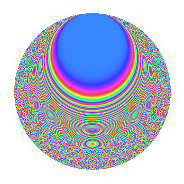# Properties

 Label 27.8.eLevel 27 Weight 8 Character orbit e Rep. character $$\chi_{27}(4,\cdot)$$ Character field $$\Q(\zeta_{9})$$ Dimension 120 Newforms 1 Sturm bound 24 Trace bound 0

# Related objects

## Defining parameters

 Level: $$N$$ = $$27 = 3^{3}$$ Weight: $$k$$ = $$8$$ Character orbit: $$[\chi]$$ = 27.e (of order $$9$$ and degree $$6$$) Character conductor: $$\operatorname{cond}(\chi)$$ = $$27$$ Character field: $$\Q(\zeta_{9})$$ Newforms: $$1$$ Sturm bound: $$24$$ Trace bound: $$0$$

## Dimensions

The following table gives the dimensions of various subspaces of $$M_{8}(27, [\chi])$$.

Total New Old
Modular forms 132 132 0
Cusp forms 120 120 0
Eisenstein series 12 12 0

## Trace form

 $$120q - 6q^{2} - 6q^{3} - 6q^{4} - 219q^{5} + 1386q^{6} - 6q^{7} - 4611q^{8} - 1728q^{9} + O(q^{10})$$ $$120q - 6q^{2} - 6q^{3} - 6q^{4} - 219q^{5} + 1386q^{6} - 6q^{7} - 4611q^{8} - 1728q^{9} - 3q^{10} + 9399q^{11} - 10545q^{12} - 6q^{13} + 16647q^{14} + 11709q^{15} + 378q^{16} - 58959q^{17} - 156051q^{18} - 3q^{19} + 240243q^{20} - 516q^{21} + 105762q^{22} - 144084q^{23} - 150894q^{24} - 107997q^{25} + 443202q^{26} + 114363q^{27} - 12q^{28} + 107886q^{29} - 179901q^{30} + 297975q^{31} - 639576q^{32} - 31338q^{33} - 265806q^{34} - 450672q^{35} + 51264q^{36} - 3q^{37} - 145596q^{38} + 1919661q^{39} + 283521q^{40} + 227085q^{41} + 3792042q^{42} - 1080969q^{43} - 1205115q^{44} - 4430925q^{45} - 3q^{46} - 5184339q^{47} - 2424039q^{48} + 976008q^{49} + 2324559q^{50} + 2918034q^{51} - 3366135q^{52} + 5631750q^{53} + 11971422q^{54} - 12q^{55} + 5254923q^{56} - 284637q^{57} + 3884295q^{58} - 12534492q^{59} - 29390886q^{60} + 3865440q^{61} - 9923646q^{62} + 3292389q^{63} - 10223619q^{64} + 17158395q^{65} + 39100653q^{66} + 5567061q^{67} + 19858455q^{68} - 12682935q^{69} - 1020993q^{70} - 18563877q^{71} - 33138540q^{72} + 3770934q^{73} - 36278085q^{74} - 26317941q^{75} - 1156998q^{76} + 8471751q^{77} + 36593658q^{78} + 16415526q^{79} + 101080590q^{80} + 45736524q^{81} - 12q^{82} + 3147699q^{83} - 27588054q^{84} - 7672131q^{85} - 70854834q^{86} - 55384731q^{87} - 34323078q^{88} - 54647613q^{89} - 25256970q^{90} + 3315066q^{91} + 73858515q^{92} + 69808065q^{93} + 22126539q^{94} + 32541447q^{95} + 33014034q^{96} + 41250117q^{97} - 88493274q^{98} - 111262167q^{99} + O(q^{100})$$

## Decomposition of $$S_{8}^{\mathrm{new}}(27, [\chi])$$ into irreducible Hecke orbits

Label Dim. $$A$$ Field CM Traces $q$-expansion
$$a_2$$ $$a_3$$ $$a_5$$ $$a_7$$
27.8.e.a $$120$$ $$8.434$$ None $$-6$$ $$-6$$ $$-219$$ $$-6$$